Midterm 2 Preparation

# Midterm 2: Version B

Find the solution set of the system graphically.

1.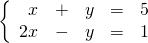For problems 2–4, find the solution set of each system by any convenient method.

1.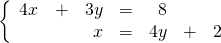2.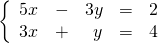3.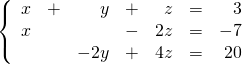Reduce the following expressions in questions 5–8.

1.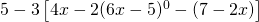2.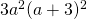3.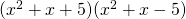4.For problems 9–12, factor each expression completely.

1.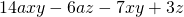2.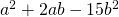3.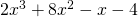4.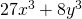Solve the following word problems.

1. The sum of the ages of a father and his daughter is 38. Six years from now, the father will be four times as old as his daughter. Find the present age of each.
2. A 90 kg mixture of two different types of nuts costs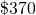. If type A costs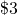per kg and type B costs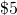per kg, how many kg of each type were used?
3. A student lab technician is combining a 10% sulfuric acid solution to 40 ml solution at 25% to dilute it to 15%.  How much of the 10% solution does the student need to add?